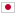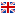Contest Duration: - (local time) (300 minutes)
E - Similarity of Subtrees/Time Limit: 2 sec / Memory Limit: 512 MB

### Problem Statement

Define the depth of a node in a rooted tree by applying the following rules recursively:

• The depth of a root node is 0.
• The depths of child nodes whose parents are with depth $d$ are $d+1$.

Let $S(T, d)$ be the number of nodes of $T$ with depth $d$. Two rooted trees $T$ and $T'$ are similar if and only if $S(T, d)$ equals $S(T', d)$ for all non-negative integer $d$.

You are given a rooted tree $T$ with $N$ nodes. The nodes of $T$ are numbered from 1 to $N$. Node 1 is the root node of $T$. Let $T_i$ be the rooted subtree of $T$ whose root is node $i$. Your task is to write a program which calculates the number of pairs $(i, j)$ such that $T_i$ and $T_j$ are similar and $i < j$.

### Input

The input consists of a single test case.

$N$
$a_1$ $b_1$

$a_{N-1}$ $b_{N-1}$

The first line contains an integer $N$ ($1 \le N \le 100{,}000$), which is the number of nodes in a tree. The following $N-1$ lines give information of branches: the $i$-th line of them contains $a_i$ and $b_i$, which indicates that a node $a_i$ is a parent of a node $b_i$. ($1 \le a_i, b_i \le N$, $a_i \ne b_i$) The root node is numbered by 1. It is guaranteed that a given graph is a rooted tree, i.e. there is exactly one parent for each node except the node 1, and the graph is connected.

### Output

Print the number of the pairs $(x,y)$ of the nodes such that the subtree with the root $x$ and the subtree with the root $y$ are similar and $x < y$.

### Sample Input 1

5
1 2
1 3
1 4
1 5

### Output for the Sample Input 1

6

### Sample Input 2

6
1 2
2 3
3 4
1 5
5 6

### Output for the Sample Input 2

2

### Sample Input 3

13
1 2
1 3
2 4
2 5
3 6
3 7
4 8
4 9
6 10
7 11
8 12
11 13

### Output for the Sample Input 3

14